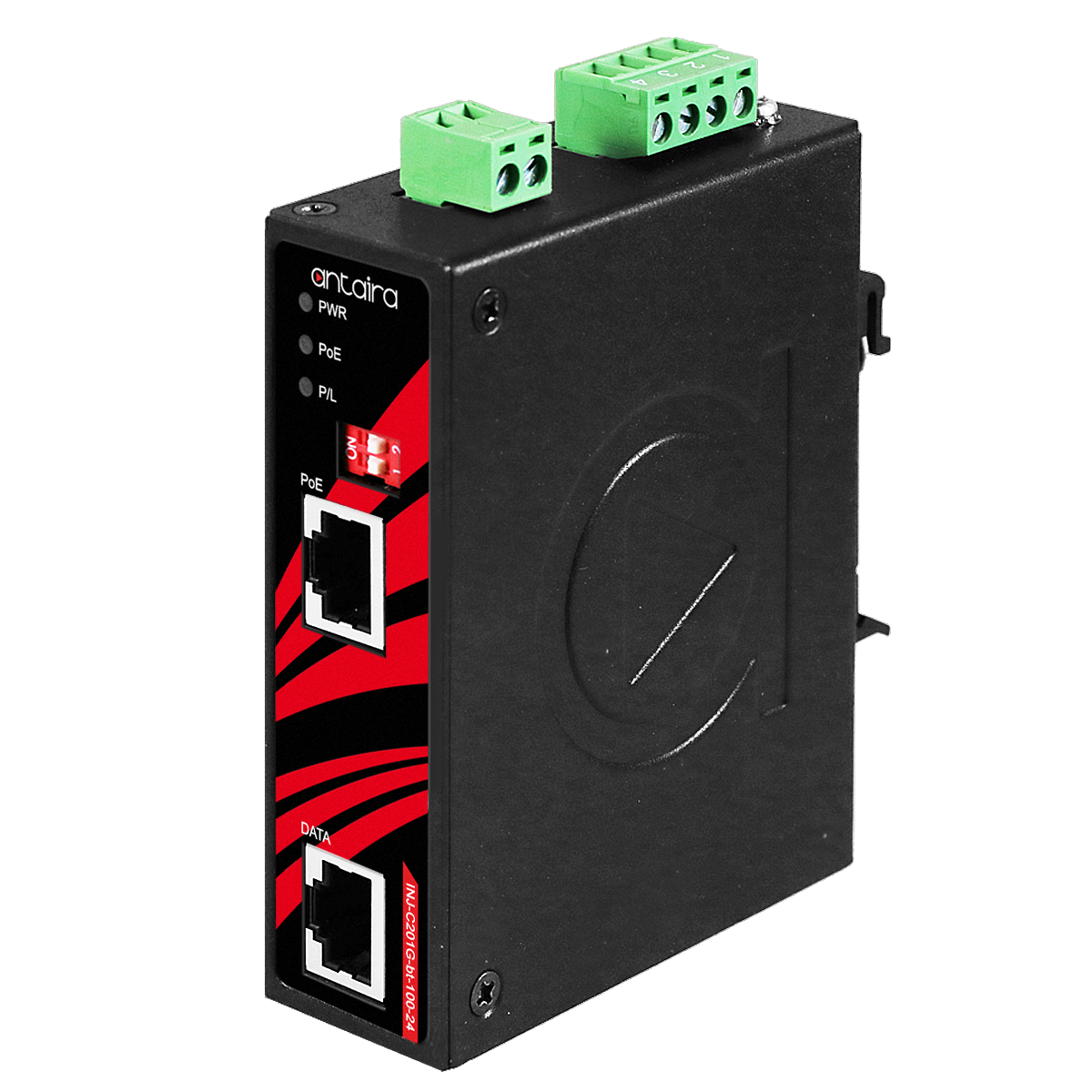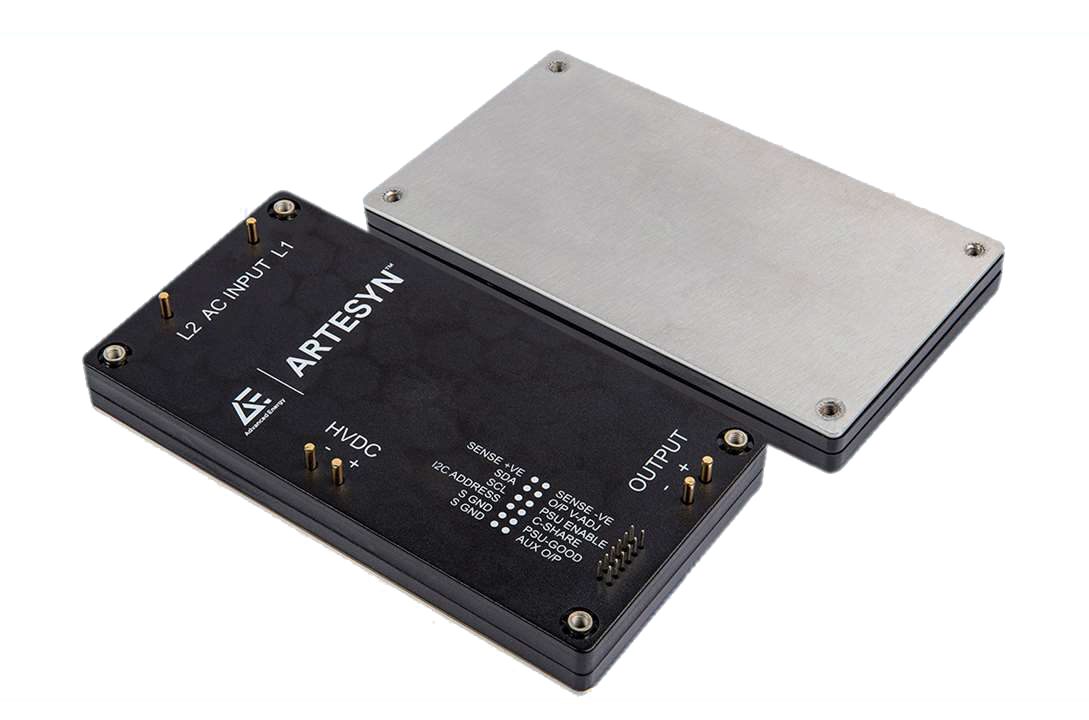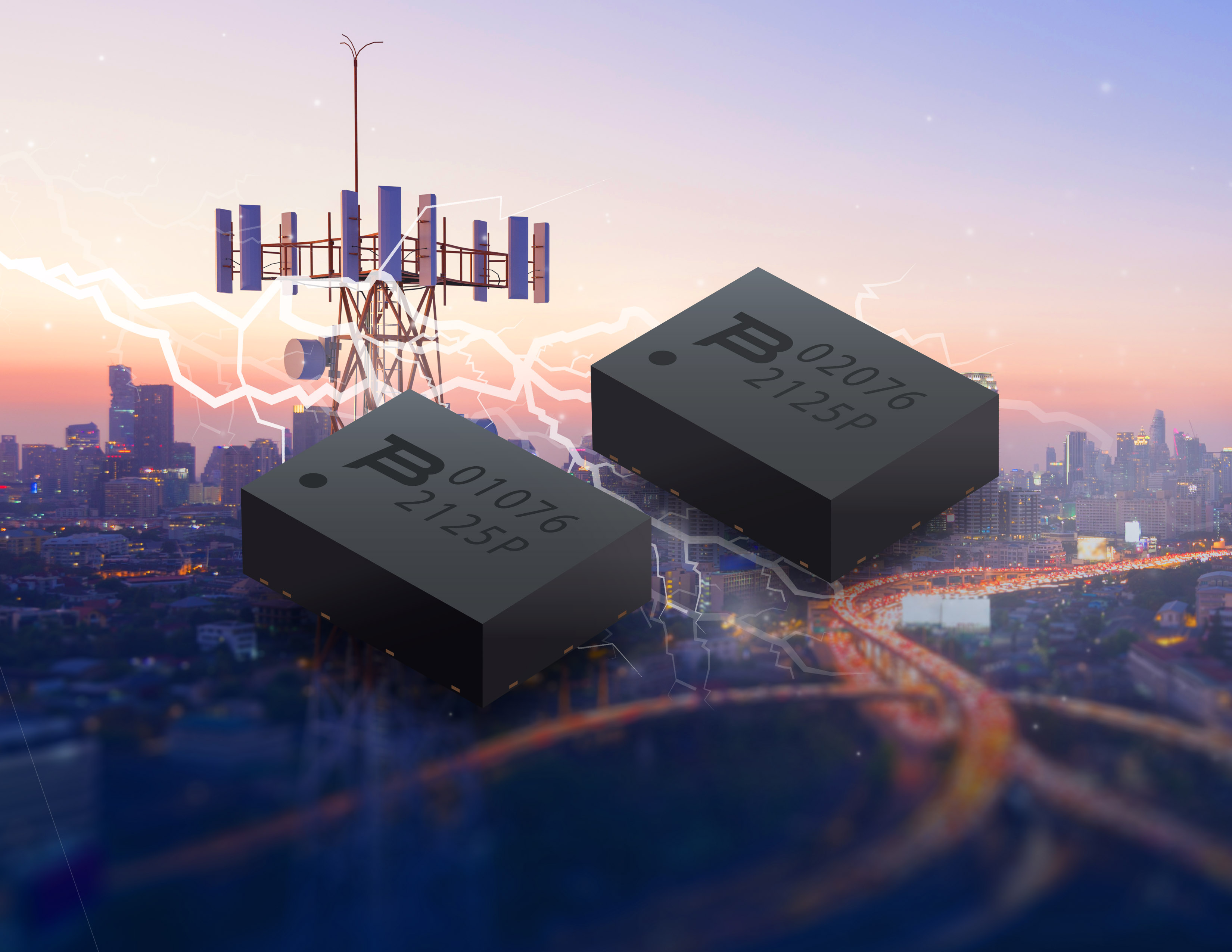Author:
Jefferson Eco and Aldrick Limjoco, Analog Devices

Date
04/15/2016PDF
##### Improper use of ferrite beads in system design can lead to some detrimental issues

An effective method for filtering high-frequency power supply noise and cleanly sharing similar voltage supply rails (i.e. analog and digital rails for mixed-signal ICs) while preserving high-frequency isolation between the shared rails is the use of ferrite beads.  A ferrite bead is a passive device that filters high frequency noise energy over a broad frequency range by becoming resistive over its intended frequency range, dissipating noise energy in the form of heat. The ferrite bead is connected in series with the power supply rail and is often combined with capacitors to ground on either side of the bead. This forms a low-pass filter network, further reducing high-frequency power supply noise.

Use with caution

Improper use of ferrite beads in system design can lead to some detrimental issues. Such examples include un-wanted resonance, due to combining the bead with decoupling capacitor for low pass filtering, and the effect of DC bias current dependency that degrades the EMI suppression capability of the bead. With proper understanding and consideration of ferrite bead behavior, these issues can be avoided.

There are important considerations that system designers need to be aware of when using ferrite beads in power supply systems such as impedance vs. frequency characteristics with varying DC bias current and unwanted LC resonance effects. Ultimately, to address the issue on the unwanted resonance, damping techniques will be introduced with a comparison of the effectiveness of each damping method will be presented.

The Analog Devices product used to demonstrate the effects of ferrite beads as output filter is a 2 A/1.2 A dc-to-dc switching regulator with independent positive and negative outputs (ADP5071). The ferrite beads used in the article are mainly chip-type surface mount packages.

Ferrite bead simplified model and simulation

A ferrite bead can be modeled as a simplified circuit consisting of resistors, an inductor, and a capacitor, as shown in Figure 1a. RDC corresponds to the dc resistance of the bead. CPAR, LBEAD, and RAC are the parasitic capacitance, the bead inductance, and the ac resistance (ac core losses) associated with the bead.

Ferrite beads are categorized by three response regions: inductive, resistive, and capacitive. These regions can be determined by looking at a ZRX plot (shown in Figure 1b), where Z is the impedance, R is the resistance, and X is the reactance of the bead. To reduce high frequency noise, the bead must be in the resistive region; this is especially desirable for electromagnetic interference (EMI) filtering applications. The component acts like a resistor, which impedes the high frequency noise and dissipates it as heat. The resistive region occurs after the bead crossover frequency (X = R) and up to the point where the bead becomes capacitive. This capacitive point occurs at the frequency where the absolute value of capacitive reactance (-X) is equivalent to R.

Click image to enlarge

Figure 1. Simplified Circuit Model (a) and Tyco Electronics BMB2A1000LN2 Measured ZRX Plot (b)

In some cases, the simplified circuit model can be used to approximate the ferrite bead impedance characteristic up to the sub-GHz range.

The Tyco Electronics BMB2A1000LN2 multilayer ferrite bead is used as an example. Figure 1b shows the measured ZRX response of the BMB2A1000LN2 for a zero dc bias current using an impedance analyzer.

For the region on the measured ZRX plot where the bead appears most inductive (Z ≈ XL; LBEAD), the bead inductance is calculated by the following equation:

where:

f is the frequency point anywhere in the region the bead appears inductive. In this example, f = 30.7 MHz

XL is the reactance at 30.7 MHz, which is 233 Ω.

Equation 1 yields an inductance value (LBEAD) of 1.208 μH.

For the region where the bead appears most capacitive (Z ≈ |XC|; CPAR), the parasitic capacitance is calculated by the following equation:

where:

f is the frequency point anywhere in the region the bead appears capacitive. In this example, f = 803 MHz

|XC| is the reactance at 803 MHz, which is 118.1 Ω.

Equation 2 yields a parasitic capacitance value (CPAR) of 1.678 pF.

The dc resistance (RDC), which is 300 mΩ, is acquired from the manufacturer data sheet. The ac resistance (RAC) is the peak impedance where the bead appears to be purely resistive. Calculate RAC by subtracting RDC from Z. Because RDC is very small compared to the peak impedance, it can be neglected. Therefore, in this case RAC is 1.082 kΩ. The ADIsimPE circuit simulator tool powered by SIMetrix/SIMPLIS was used to generate the impedance vs. the frequency response. Figure 2a shows the circuit simulation model with the calculated values and Figure 2b shows both the actual measurement and simulated result. In this example, the impedance curve from the circuit simulation model closely matches the measured one.

Click image to enlarge

Figure 2. Circuit Simulation Model (a) and Actual Measurement vs. Simulation (b)

The ferrite bead model can be useful in noise filtering circuit design and analysis. For example, approximating the inductance of the bead can be helpful in determining the resonant frequency cut-off when combined with a decoupling capacitor in a low-pass filter network. However, the circuit model specified in this article is an approximation with a zero dc bias current. This model may change with respect to dc bias current, and in other cases, a more complex model is required.

DC bias current considerations

Selecting the right ferrite bead for power applications requires careful consideration not only of the filter bandwidth, but also of the impedance characteristics of the bead with respect to dc bias current. In most cases, manufacturers only specify the impedance of the bead at 100 MHz and publish data sheets with frequency response curves at zero dc bias current. However, when using ferrite beads for power supply filtering, the load current going through the ferrite bead is never zero, and as dc bias current increases from zero, all of these parameters change significantly.

As the dc bias current increases, the core material begins to saturate, which significantly reduces the inductance of the ferrite bead. The degree of inductance saturation differs depending on the material used for the core of the component. Figure 3a shows the typical dc bias dependency of the inductance for two ferrite beads. With 50% of the rated currents, the inductance decreases by up to 90%.

For effective power supply noise filtering, use ferrite beads at about 20% of their rated dc current. As shown in these two examples, the inductance at 20% of the rated current drops to about 30% for the 6 A bead and to about 15% for the 3 A bead. The current rating of ferrite beads is an indication of the maximum current the device can take for a specified temperature rise and not a real operating point for filtering purposes.

In addition, the effect of dc bias current can be observed in the reduction of impedance values over frequency, which in turn reduces the effectiveness of the ferrite bead and its ability to remove EMI. Figure 3b and Figure 3c show how the impedance of the ferrite bead varies with dc bias current. By applying just 50% of the rated current, the effective impedance at 100 MHz dramatically drops from 100 Ω to 10 Ω for the TDK MPZ1608S101A (100 Ω, 3 A, 0603) and from 70 Ω to 15 Ω for the Wurth Elektronik 742 792 510 (70 Ω, 6 A, 1812).

Click image to enlarge

Figure 3. (a) Effect of DC Bias on Bead Inductance, (b) TDK MPZ1608S101A and (c) Wurth Elektronik 742 791 510 Impedance Curves with Respect to DC Bias Current

System designers must be fully aware of the effect of dc bias current on bead inductance and effective impedance, as this can be critical in applications that demand high supply current.

LC resonance effect

Resonance peaking is possible when implementing a ferrite bead together with a decoupling capacitor. This commonly overlooked effect can be detrimental because it may amplify ripple and noise in a given system instead of attenuating it. In many cases, this peaking occurs around popular switching frequencies of DC/DC converters.

Peaking occurs when the resonant frequency of a low-pass filter network, formed by the ferrite bead inductance and the high Q decoupling capacitance, is below the crossover frequency of the bead. The resulting filter is underdamped. Figure 4a shows the measured impedance vs. frequency plot of the TDK MPZ1608S101A. The resistive component, which is depended upon to dissipate unwanted energy, does not become significant until about 20 MHz to 30 MHz. Below this frequency, the ferrite bead still has a very high Q and acts like an ideal inductor. LC resonant frequencies for typical bead filters are generally in the 0.1 MHz to 10 MHz range. For typical switching frequencies in the 300 kHz to 5 MHz range, additional damping is required to reduce the filter Q.

As an example of this effect, Figure 4b shows the S21 frequency response of the bead and capacitor low-pass filter, which displays a peaking effect. The ferrite bead used is a TDK MPZ1608S101A (100 Ω. 3 A, 0603) and the decoupling capacitor used is a Murata GRM188R71H103KA01 low ESR ceramic capacitor (10 nF , X7R, 0603). Load current is in the microampere range.

Click image to enlarge

Figure 4. TDK MPZ1608S101A ZRX Plot (a) and S21 Response for Ferrite bead and Capacitor Low-Pass Filter( b)

In addition, signal gain can be seen from 1 MHz to 3.5 MHz. This peaking is problematic if it occurs in the frequency band in which the switching regulator operates. This amplifies the unwanted switching artifacts, which can wreak havoc on the performance of sensitive loads such as the phased-lock loop (PLL), voltage-controlled oscillator (VCO), and high resolution analog-to-digital converter (ADC).

The result shown in Figure 4b has been taken with a very light load (in the microampere range), but this is a realistic application in sections of circuits that need just a few microamperes to 1 mA of load current or sections that are turned off to save power in some operating modes. This potential peaking creates additional noise in the system that can create unwanted crosstalk.

As an example, Figure 5 shows an ADP5071 application circuit with an implemented bead filter and Figure 6 shows the spectral plot at the positive output. The switching frequency is set at 2.4 MHz, the input voltage is 9 V, the output voltage is set at 16 V, and the load current of 5 mA.

Click image to enlarge

Figure 5. ADP5071 Application Circuit with Bead and Capacitor Low-Pass Filter Implementation on Positive Output

Click image to enlarge

Resonant peaking occurs at around 2.5 MHz due to the inductance of the bead and the 10 nF ceramic capacitor. Instead of attenuating the fundamental ripple frequency at 2.4 MHz, a gain of 10 dB occurs. Other factors that have an effect on the resonant peaks are the series and load impedances of the ferrite bead filter. Peaking significantly reduced and dampen for higher source resistance. However, the load regulation degrades with this approach, making it unrealistic in practice. The output voltage droops with load current due to the drop from the series resistance. Load impedance also affects the peaking response. Peaking is worst for light load conditions.

Damping methods

This section describes three damping methods that a system engineer can use to reduce the level of resonant peaking significantly (see Figure 7).

Click image to enlarge

Figure 7. Actual Frequency Response for Various Damping Methods

Method A consists of adding a series resistor to the decoupling capacitor path which dampens the resonance of the system but degrades the bypass effectiveness at high frequencies.

Method B consists of adding a small parallel resistor across the ferrite bead which also dampens the resonance of the system. However, the attenuation characteristic of the filter is reduced at high frequencies.

Figure 8 shows the impedance vs. frequency curve of the MPZ1608S101A with and without a 10 Ω parallel resistor. The orange dashed curve is the overall impedance of the bead with a 10 Ω resistor in parallel. The impedance of the bead and resistor combination is significantly reduced and is dominated by the 10 Ω resistor. However, the 3.8 MHz crossover frequency for the bead with the 10 Ω parallel resistor is much lower than the crossover frequency of the bead on its own at 40.3 MHz. The bead appears resistive at a much lower frequency range, lowering the Q for improved damped performance.

Click image to enlarge

Figure 8. MPZ1608S101A ZRX Plot (a) and  MPZ1608S101A ZRX Plot, Zoom View (b)

Method C consists of adding a large capacitor (CDAMP) with a series damping resistor (RDAMP), which is often optimum. Adding the capacitor and resistor dampens the resonance of the system and does not degrade the bypass effectiveness at high frequencies. Implementing this method avoids excessive power dissipation on the resistor due to a large dc blocking capacitor. The capacitor must be much larger than the sum of all decoupling capacitors, which reduces the required damping resistor value. The capacitor impedance must be sufficiently smaller than the damping resistance at the resonant frequency to reduce the peaking.

Figure 9 shows the ADP5071 positive output spectral plot with Method C damping implemented on the application circuit shown in Figure 5. The CDAMP and RDAMP used are a 1 μF ceramic capacitor and a 2 Ω SMD resistor, respectively. The fundamental ripple at 2.4 MHz is reduced by 5 dB as opposed to the 10 dB gain shown in Figure 9.

Click image to enlarge

Figure 9. ADP5071 Spectral Output plus Bead and Capacitor Low-Pass Filter with Method C Damping

Generally, Method C is the most elegant and is implemented by adding a resistor in series with a ceramic capacitor rather than buying an expensive dedicated damping capacitor. The safest designs always include a resistor that can be tweaked during prototyping and that can be eliminated if not necessary. The only drawbacks are the additional component cost and greater required board space.

### RELATED#### PoE Injectors Bring Plug-and-Play Power to ﻿Industrial Network Devices

Aug 8,2022#### AC/DC Board-Mounted Converter Simplifies Reliable Operation

Aug 4,2022#### Gigabit Fiber-to-Ethernet Media Converters Ideal for Long Distance, High Bandwidth Connectivity

Aug 2,2022Aug 2,2022